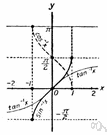inverse function

(redirected from Invertible function)
Also found in: Thesaurus, Encyclopedia.
Related to Invertible function: Invertible matrix, Inverse functions

inverse function

n. Mathematics
A function whose relation to a given function is such that their composite is the identity function. It is often found by interchanging dependent and independent variables.

in′verse func′tion

n.
Math. the function that replaces another function when the dependent and independent variables of the first function are interchanged for an appropriate set of values of the dependent variable.
[1810–20]
 Noun 1inverse function - a function obtained by expressing the dependent variable of one function as the independent variable of another; f and g are inverse functions if f(x)=y and g(y)=xfunction, mapping, mathematical function, single-valued function, map - (mathematics) a mathematical relation such that each element of a given set (the domain of the function) is associated with an element of another set (the range of the function)